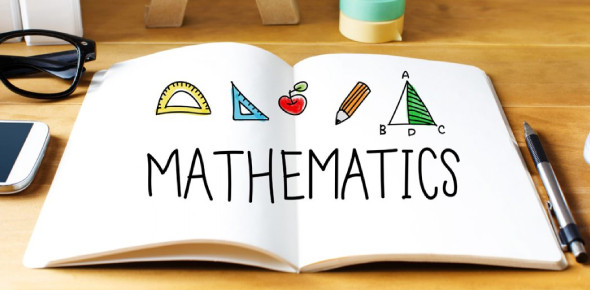# GK: Math Practice Quiz!

15 Questions | Total Attempts: 443SettingsNot everyone can answer any mathematical question brought to them, but the ones that can are always placed first to tutor other people and can only do this through practice. Brush up on your knowledge about general knowledge math with these quiz questions and score a perfect A.

• 1.
What is the shape where its angles add up to 720 degrees?
• A.

Diamond

• B.

Triangle

• C.

Hexagon

• D.

Rhombus

• 2.
Two angles, measuring 58 degrees and 32 degrees, would be considered what kind of angles?
• A.

Vertical Angles

• B.

Complementary Angles

• C.

90 Degrees

• D.

Supplementary Angles

• 3.
What shape has 2 parallel sides and 2 perpendicular sides, angles adding up to 360 degrees?
• A.

Cylinder

• B.

Rectangle

• C.

Trapezoid

• D.

Polygon

• 4.
What is the average weight loss for four men who dropped 55, 36, 15 and 74 pounds each?
• A.

45

• B.

18

• C.

68

• D.

80

• 5.
If g(x) = x/4, what is g(1/4)?
• A.

1/256

• B.

4

• C.

4-Jan

• D.

16-Jan

• 6.
What is the probability of rolling less than a 4 when tossing a single die?
• A.

3-Feb

• B.

4-Jan

• C.

3-Jan

• D.

2-Jan

• 7.
How many ways can a group of 7 choose a committee of 4?
• A.

1

• B.

840

• C.

210

• D.

35

• 8.
If m divided by 6 has 5 as a remainder, what is the remainder if 4m is divided by 6?
• A.

5

• B.

0

• C.

2

• D.

1

• 9.
If 3x - x = 2x + x + 20, then x = ?
• A.

0

• B.

-5

• C.

-20

• D.

20

• 10.
If a + b = 40 and a < 15, which of the following must be true?
• A.

B > 25

• B.

B < 25

• C.

A > 0

• D.

B < 40

• 11.
If 50,505 + x = 55,555, then 50,505 - 10x = ?
• A.

0

• B.

5

• C.

-50.5

• D.

5.05

• 12.
Which fraction is equal to 2/3 + 4/5 + 1/2?
• A.

87/60

• B.

59/30

• C.

49/30

• D.

59/15

• 13.
If your Canadian uncle tells you he got a ticket for driving 103 km/h, how fast was he going?
• A.

64 MPH

• B.

72 MPH

• C.

79 MPH

• D.

103 MPH

• 14.
If a two pound bag of grass seed covers 720 square feet, how many bags are needed to seed 1 acre?
• A.

112

• B.

47

• C.

60.5

• D.

89.3

• 15.
If two lines both have a slope of 1/3 and they don't share any points, what are they called?
• A.

Perpendicular

• B.

Same Line

• C.

Intersecting

• D.

Parallel

Related TopicsBack to top